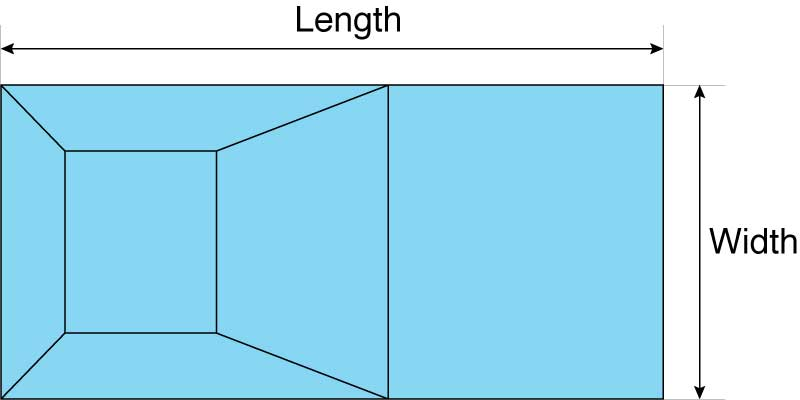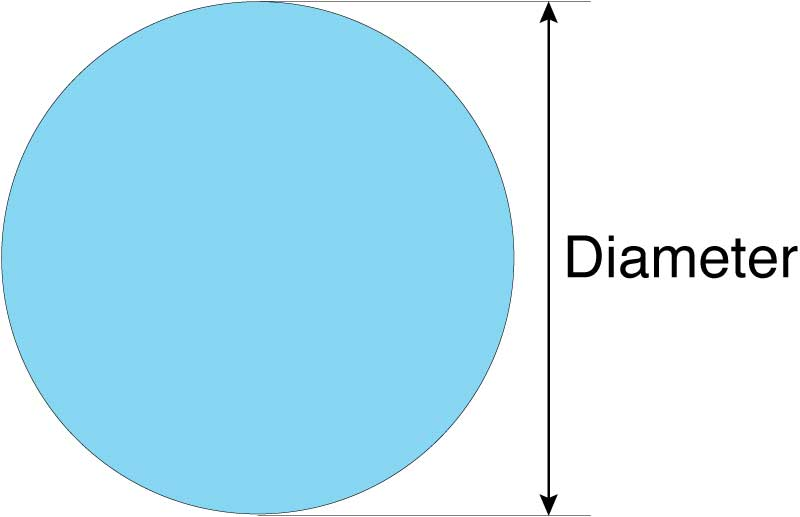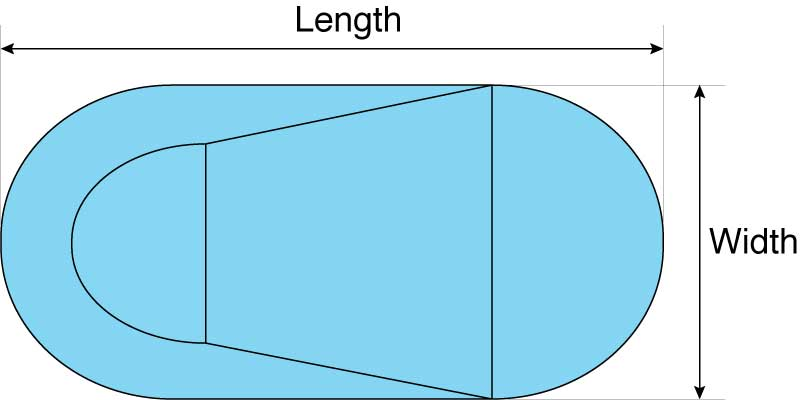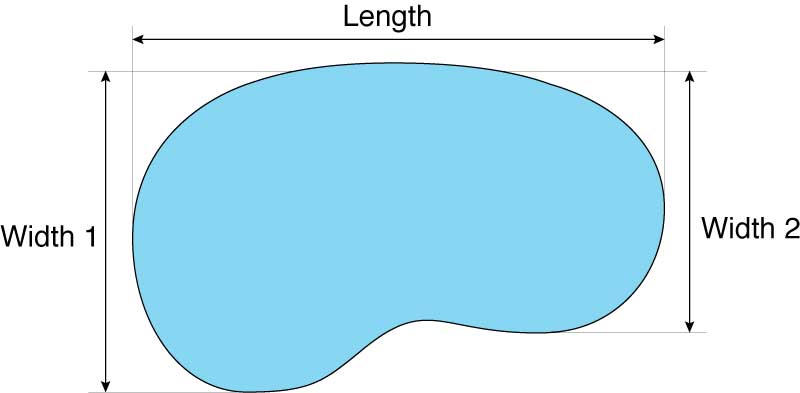# Pool Volume Calculator

Calculate how many gallons or liters of water are needed to fill a swimming pool by selecting the pool shape and size below.

Pool Shape:

## Results:

Gallons

Liters

Cubic Feet
Learn how we calculated this below

## How to Calculate Pool Volume

If you’re here, then there’s a good chance you’re trying to find the size of your pool in gallons or trying to figure out how much water is needed to fill it. Keep reading to see the volume formulas for various pool shapes.

### How to Calculate Rectangular Pool VolumeYou can find the volume of a rectangular swimming pool in gallons by multiplying the length by the width by the average depth in feet by 7.48. The formula to find pool volume in gallons is thus:

volume = length × width × depth × 7.48

The first part of this formula determines the volume of water in cubic feet. The second part converts cubic feet to gallons; there are 7.48 gallons of water per cubic foot of water.

Most pools have varying depths that slope from shallow to deeper. To accurately find the volume of water, you’ll need to estimate the average depth. To find the average depth add the shallow depth with the deep depth, then divide by 2, e.g. (shallow + deep) ÷ 2.

For instance, if your pool’s deep end is 12 feet and the shallow end is 4 feet, your average depth will be 8 feet.

If you have an L, T, or stepped-shaped pool, you can use the same basic formula to calculate your volume. You will need to treat each section of the pool separately, then add them together at the end for the total volume.

For example, if your L-shaped pool has one section that is 20 feet long and 10 feet wide with an average depth of 8 feet, and a second section that is 10 feet long and 10 feet wide, with an average depth of 6 feet, you will calculate the volume like this:

20′ × 10′ × 8′ × 7.48 = 11,968 gallons
10′ × 10′ × 6′ × 7.48 = 4,680 gallons
11,968 + 4,680 = 16,648 total gallons

If your pool is triangular in shape or has a triangular section, the triangular area can be found by multiplying length × width and then multiplying the result by 1/2.

For example, you can calculate the number of gallons for a triangular shaped pool that has a length of 20 feet and a width of 10 feet with an average depth of 6 feet will have a formula to calculate the number of gallons like this:

20′ × 10′ × 1/2 × 6′ × 7.48 = 4,488 gallons

### How to Calculate Circular Pool VolumeYou can calculate the volume of a circular swimming pool by multiplying 3.14 times the radius squared times 7.48. Thus, the formula is:

volume = πr2 × average depth × 7.48

The first part of this formula (πr2) determines the area, and multiplying by the depth finds the volume. Multiplying by 7.48 finds the gallons of water per cubic foot of water.

To get the radius squared, multiply 5 feet by 5 feet, giving you 25 feet. If your average depth is 4 feet, then your volume formula will look like:

3.14(π) × 25′(r2) × 4′ × 7.48 = 2,349 gallons

### How to Calculate Oval Pool VolumeYou can calculate the volume of an oval swimming pool by multiplying the length times the width in feet times 3.14 divided by 4 times the average depth in feet times 7.48. The formula is:

volume = length × width × π ÷ 4 × depth × 7.48

The first part of this formula (length × width × π ÷ 4) determines the area, and multiplying by the depth finds the volume. Again, multiplying by 7.48 determines how many gallons of water are in the pool.

Like a rectangular pool, the average depth can be found by adding the shallow and deep depths and then dividing by 2.

### How to Calculate Irregular Oblong or Kidney-Shaped Pool VolumeCalculating the volume of an oblong or kidney-shaped pool is a little more complicated. Begin by getting the total length of the pool, and the width of both its ends.

You will add together the width of both ends, so if your pool has an end that is 10 feet wide and an end that is 6 feet wide, you will have a total width of 16 feet for the purposes of this calculation.

Calculate the volume of the pool by multiplying the length in feet times your total, added width (the shorter width in feet + the longer width in feet) times 0.45 times the average depth in feet times 7.48.

volume = length × (short width + the long width) × 0.45 × depth × 7.48

The first part (length × (short width + long width) × 0.45) is used to find the area, and then the volume is found by multiplying by the depth. Like before, multiplying by 7.48 finds the number of gallons in the pool.

For example, for a pool that is 20 feet long with an added width of 18 feet and an average depth of 8 feet, your formula will look like:

20′ × 18′ × 0.45 × 8′ × 7.48 = 9,694 gallons

## Weights and Measures

To find pool volume in liters, you can use our gallons to liters unit conversion calculator or multiply the gallons by 3.79 – the number of liters in one gallon.

To find the weight in pounds of the water in the pool, you can multiply the number of gallons by 8.33, or use our water weight calculator.

One cubic foot of water is 12″ wide × 12″ long × 12″ deep and weighs 62.4 pounds.